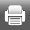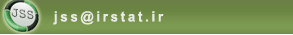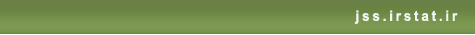[Home ] [Archive]   [ فارسی ]Back to the articles list Back to browse issues page
Smallest Confidence Region for the Mean and Standard Deviation of Two-Parameter Uniform Distribution
Abstract:   (337 Views)
In this paper, based on an appropriate pivotal quantity, two methods are introduced to determine confidence region for the mean and standard deviation in a two parameter uniform distribution, in which the application of numerical methods is not mandatory. In the first method, the smallest region is obtained by minimizing the confidence regionchr('39')s area, and in the second method, a simultaneous Bonferroni confidence interval is introduced by using the smallest confidence intervals. By the comparison of area and coverage probability of the introduced methods, as well as, comparison of the width of strip including the standard deviation in both methods, it has been shown that the first method has a better efficiency. Finally, an approximation for the quantile of F
distribution used in calculating the confidence regions in a special case is presented.
Keywords: Bonferroni Method, Joint Confidence Region, Two-Parameter Uniform Distribution.
Type of Study: Applied | Subject: Statistical Inference